Jet Set Go! All about Aeroplanes Jet Set Go! All about Aeroplanes

# Circle Theorem

Circle theorem includes the concept of tangents, sectors, angles, the chord of a circle and proofs. A circle is the locus of all points in a plane which are equidistant from a fixed point. The fixed point is called the centre of the circle, and the constant distance between any point on the circle and its centre is called the radius. The perimeter of a circle is known as the circumference and the area occupied by a circle in a plane is its area. The tangent is perpendicular to the radius, at any point of a circle, through the point of contact. Let us learn more about the circle and its theorems here.

## What is the Chord of a Circle?

A line segment, which joins any two points on the circle’s circumference, is known as a chord of the circle. Diameter is the largest chord which passes through the centre of the circle.

Let us consider a circle, which has AB as diameter, CD is the chord of the circle and OE is the radius. See the figure below.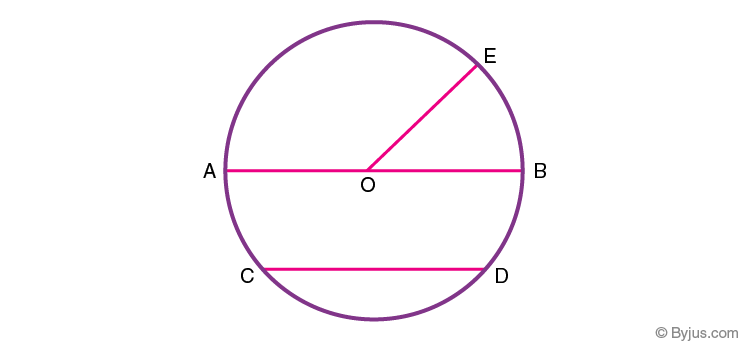Let us see the different circle theorems.

## Circles Theorem Class 9

In Class 9, students will come across the basics of circles. Here, we will learn different theorems based on the circle’s chord. The theorems will be based on these topics:

• Angle Subtended by a Chord at a Point
• The perpendicular from the Centre to a Chord
• Equal Chords and their Distances from the Centre
• Angle Subtended by an Arc of a Circle

Now let us learn all the circle theorems and proofs.

## Circle Theorems and Proofs

### Theorem 1:

“Two equal chords of a circle subtend equal angles at the centre of the circle.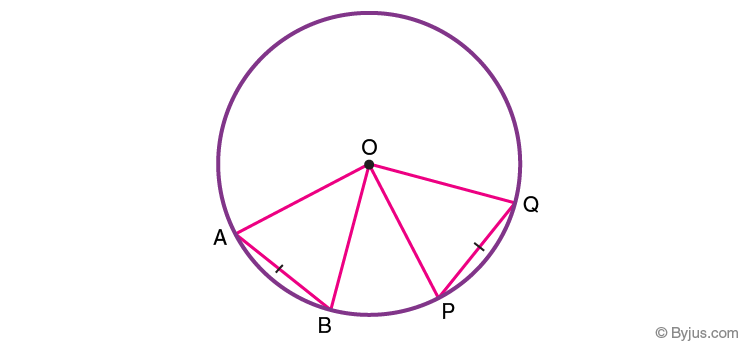Proof: Given, in ∆AOB and ∆POQ,

AB = PQ (Equal Chords) …………..(1)

OA = OB= OP=OQ (Radii of the circle) ……..(2)

From eq 1 and 2, we get;

∆AOB ≅ ∆POQ (SSS Axiom of congruency)

Therefore, by CPCT (corresponding parts of congruent triangles), we get;

∠AOB = ∠POQ

Hence, Proved.

### Converse of Theorem 1:

“If two angles subtended at the center by two chords are equal, then the chords are of equal length.”

Proof: Given, in ∆AOB and ∆POQ,

∠AOB = ∠POQ (Equal angle subtended at centre O) ……………(1)

OA = OB = OP = OQ (Radii of the same circle) ……………(2)

From eq. 1 and 2, we get;

∆AOB ≅ ∆POQ (SAS Axiom of congruency)

Hence,

AB = PQ (By CPCT)

### Theorem 2:

“The perpendicular to a chord bisects the chord if drawn from the centre of the circle.”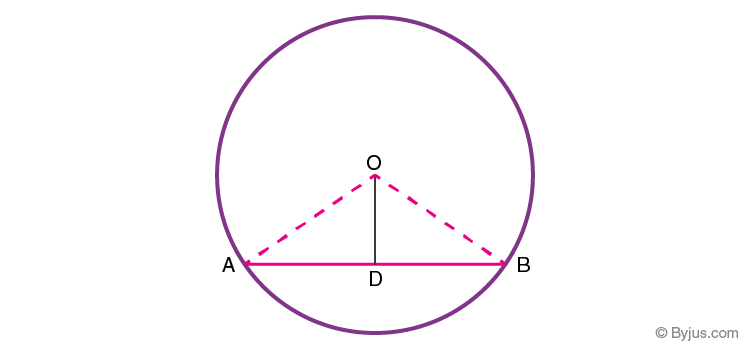In the above figure, as per the theorem, OD ⊥ AB, therefore, AD = DB.

Proof: Given, in ∆AOD and ∆BOD,

∠ADO = ∠BDO = 90° (OD ⊥ AB) ………(1)

OA = OB (Radii of the circle) ……….(2)

OD = OD (Common side) ………….(3)

From eq. (1), (2) and (3), we get;

∆AOB ≅ ∆POQ (R.H.S Axiom of congruency)

Hence, AD = DB (By CPCT)

### Converse of Theorem 2:

“A straight line passing through the centre of a circle to bisect a chord is perpendicular to the chord.”

Proof: Given, in ∆AOD and ∆BOD,

AD = DB (OD bisects AB) ………….(1)

OA = OB (Radii of the circle) ………….(2)

OD = OD (Common side) …………..(3)

From eq. 1, 2 and 3, we get;

∆AOB ≅ ∆POQ (By SSS Axiom of congruency)

Hence,

∠ADO = ∠BDO = 90° (By CPCT)

### Theorem 3:

“Equal chords of a circle are equidistant (equal distance) from the centre of the circle.”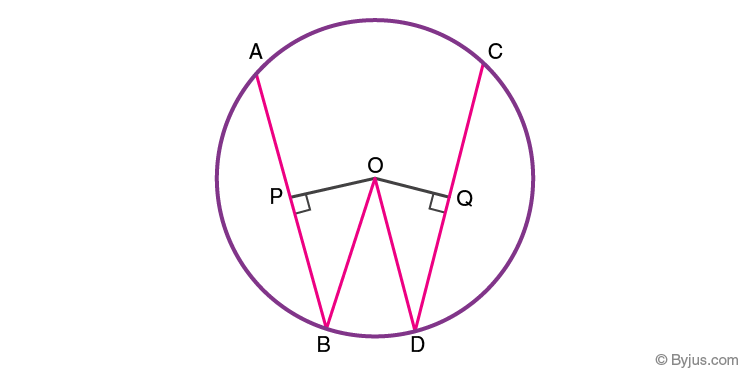Construction: Join OB and OD

Proof: Given, In ∆OPB and ∆OQD

BP = 1/2 AB (Perpendicular to a chord bisects it) ……..(1)

DQ = 1/2 CD (Perpendicular to a chord bisects it) ……..(2)

AB = CD (Given)

BP = DQ (from eq 1 and 2)

OB = OD (Radii of the same circle)

∠OPB = ∠OQD = 90° (OP ⊥ AB and OQ ⊥ CD)

∆OPB ≅ ∆OQD ( By R.H.S Axiom of Congruency)

Hence,

OP = OQ ( By CPCT)

### Converse of Theorem 3:

“Chords of a circle, which are at equal distances from the centre are equal in length, is also true.”

Proof: Given, in ∆OPB and ∆OQD,

OP = OQ ………….(1)

∠OPB = ∠OQD = 90° ………..(2)

OB = OD (Radii of the same circle) ………..(3)

Therefore, from eq. 1, 2 and 3, we get;

∆OPB ≅ ∆OQD (By R.H.S Axiom of Congruency)

BP = DQ ( By CPCT)

1/2 AB = 1/2 CD (Perpendicular from center bisects the chord)

Hence,

AB = CD

### Theorem 4:

“Measure of angles subtended to any point on the circumference of the circle from the same arc is equal to half of the angle subtended at the center by the same arc.”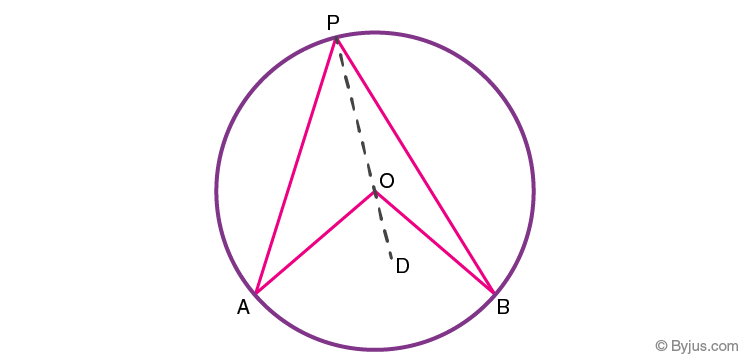From the above figure,

∠AOB = 2∠APB

Construction: Join PD passing through centre O

Proof: In ∆AOP,

OA = OP (Radii of the same circle) ………..(1)

∠OAP = ∠OPA (Angles opposite to equal sides of a triangle) …………(2)

∠AOD = ∠OAP + ∠OPA (Exterior Angle Property of the triangle) …………(3)

Hence, from eq. 2 and 3 we get;

∠AOD = 2∠OPA ………….(4)

Similarly in ∆BOP,

Exterior angle, ∠BOD = 2 ∠OPB …………(5)

∠AOB = ∠AOD + ∠BOD

From eq. 4 and 5, we get;

⇒ ∠AOB = 2∠OPA + 2∠OPB

⇒ ∠AOB = 2(∠OPA + ∠OPB)

⇒ ∠AOB = 2∠APB

Hence, proved.

### Theorem 5:

“The opposite angles in a cyclic quadrilateral are supplementary.”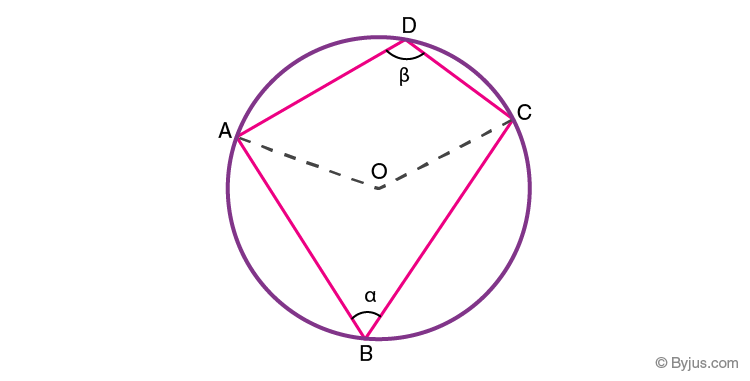Proof:

Suppose, for arc ABC,

∠AOC = 2∠ABC = 2α (Theorem 4) …………….(1)

Reflex ∠AOC = 2 ∠ADC = 2β (Theorem 4) …………..(2)

∠AOC + Reflex ∠AOC = 360°

From eq. 1 and 2, we get;

⇒ 2 ∠ABC + 2∠ADC = 360°

⇒ 2α + 2β = 360°

⇒α + β = 180°

## Video Lessons on Circles

### Introduction to Circles### Parts of a Circle### Area of a Circle